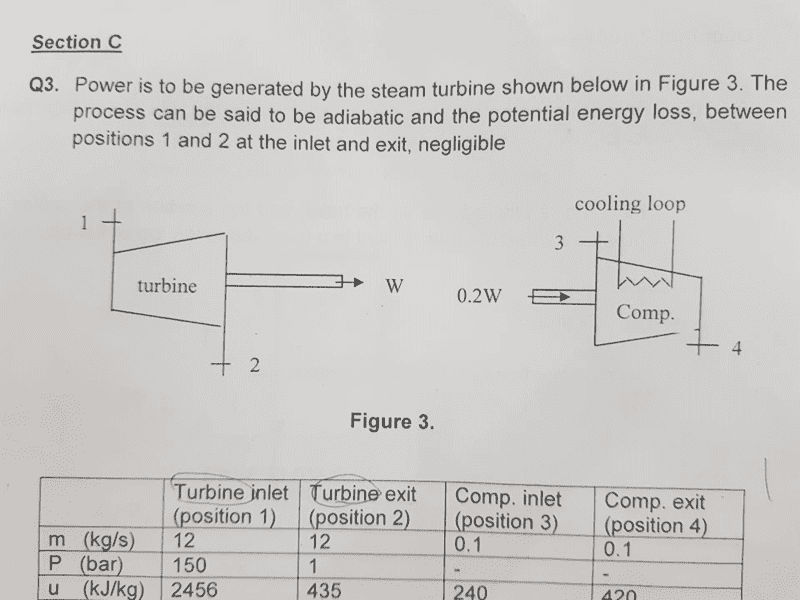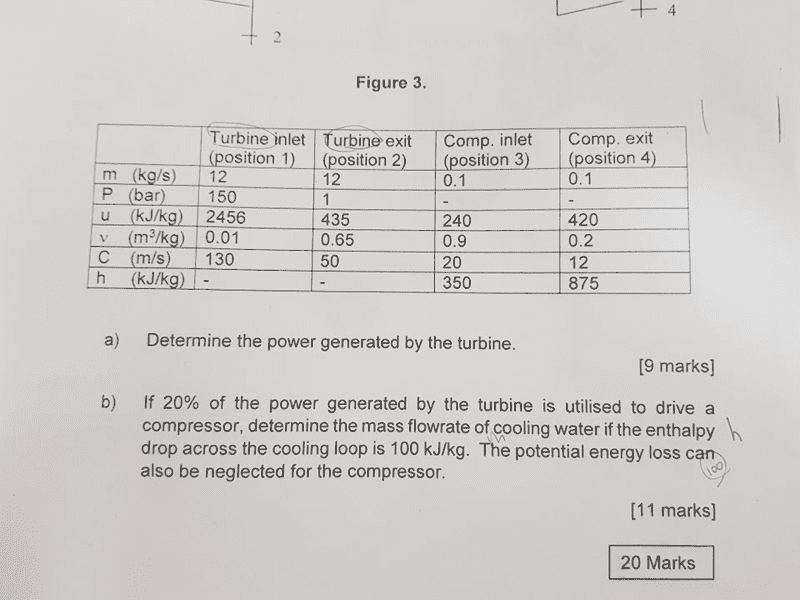# Power generated by the steam turbine

• Joon
In summary, the conversation involves a person seeking help with a physics problem in an online forum. They are directed to the homework section and given guidance on how to solve the problem using the first law of thermodynamics for open systems. The concept of external energy is also clarified.

#### Joon

Moved from a technical forum, no template.
Hello,#### Attachments

Hi,

first of all, your question seems to be homework or preparation for an exam, therefore you should have asked it in the homework's section using the template (so don't be surprised if it will be moved). Secondly, to get help for homework, the guidlines demand to show a real attempt to solve the problem by yourself, therefore please show us what you gave done so far (and have a look at the homework template).

However, I suppose you can assume the steady state operation of the turbine (and the compressor). You'll need the first law of thermodynamics for open systems.

•Chestermiller
Oh my bad, I'm new to Physicsforums.
Where can I find the homework section?

For this question, I'm not really sure where to start. That's why I couldn't show what I had done.
I know the equation for work when the process is adiabatic but it didn't help me much.
It would be nice if you could give several steps to start with, then I might be able to solve on my own.
Thanks.

I've found the homework section, thanks.

Joon said:
Oh my bad, I'm new to Physicsforums.
Where can I find the homework section?

Just click the link "FORUMS" in the header, the first block contains the homework section (template included, when you start a thread there).

Joon said:
For this question, I'm not really sure where to start.

You need the first law of thermodynamics for open systems, since mass enters/leaves the turbine it is not a closed system. The law reads

$$\delta Q +\delta W + \sum \left[\dot{m}_i \left(e_i+h_i \right] \right) = \Delta U + \Delta E$$,

##Q## ... heat
##W## ... work
##h## ... specific enthalpy
##e## ... specific external energy
##U## ...internal energy
##E## ... external energy

Now you can simply the formula with respect to your problem (i.e. which terms are equals zero?). Start with that, then you will see which data you need from the table.

stockzahn said:
Just click the link "FORUMS" in the header, the first block contains the homework section (template included, when you start a thread there).

You need the first law of thermodynamics for open systems, since mass enters/leaves the turbine it is not a closed system. The law reads

$$\delta Q +\delta W + \sum \left[\dot{m}_i \left(e_i+h_i \right] \right) = \Delta U + \Delta E$$,

##Q## ... heat
##W## ... work
##h## ... specific enthalpy
##e## ... specific external energy
##U## ...internal energy
##E## ... external energy

Now you can simply the formula with respect to your problem (i.e. which terms are equals zero?). Start with that, then you will see which data you need from the table.
I think by external energy you mean, in more familiar terminology, the sum of gravitational potential energy and kinetic energy.

Chestermiller said:
I think by external energy you mean, in more familiar terminology, the sum of gravitational potential energy and kinetic energy.

Yes, I do. Is exernal energy the wrong or an uncommon expression? Probably I should look up the technical vocabulary ...